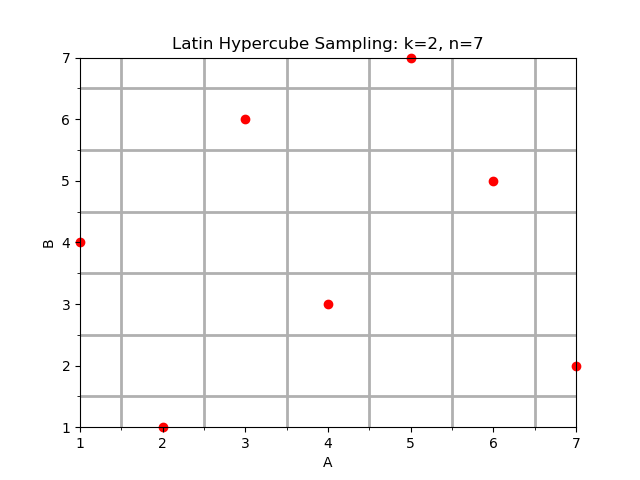# Latin Hypercube (Stat-Ease 360® only)Above is an example of a Latin Hypercube design for $$k = 2$$ factors and $$n = 7$$ runs (levels).

Latin Hypercube designs are model independent, space filling designs often used in computer experiments. In these designs each of the $$k$$ factors is divided into $$n$$ equal levels such that there is only one run containing a given level of a factor. The number of total runs is also equal to $$n$$.

Presently these designs are optimized by distance in order fill out the factor space. The integer levels are converted to the -1 to +1 coded space and subsequently the space of actual factor values.

Numeric Factors: The number of continuous numeric factors in the Latin hypercube design.

Runs: The number of runs $$n$$ in the design and the number of levels for each numeric factor.

References

• Brian Beachkofski and Ramana Grandhi. Improved Distributed Hypercube Sampling, pages 1–7. Number 1274. American Institute of Aeronautics and Astronautics, 2002.

• M.D. McKay, R.J. Beckman, and W.J. Conover. A comparison of three methods for selecting values of input variables in the analysis of output from a computer code. Technometrics, 21(2):239–245, 1979.

• Michael Stein. Large sample properties of simulations using latin hypercube sampling. Technometrics, 29(2):143–151, 1987.